# Physics Flashcards - DC and AC Circuits

The flashcards below were created by user awc1990 on FreezingBlue Flashcards.

1. (T/F) In DC current, the charge flows in one direction only.
True.
2. (T/F) In AC current, the charge flows in one direction only.
False, in AC current, the flow of charge changes direction periodically.
3. (T/F) By convection, the direction of current is the direction in which a negative charge would flow.
False, the direction of current is the direction in which a positive charge would flow.
4. What is the formula for Ohm's Law?

V =
Volts = (current)(resistance) = iR
5. How do the following affect the resistance of a conductor?

1. Increased length

2. Increased cross-sectional area

3. Increased temperature

4. Increased resistivity (ρ)
1. As length increases, so does resistance.

2. As cross-sectional area increases, resistance decreases.

3. As temperature increases, resistance typically increases.

4. As resistivity increases so does resistance.
6. What is the formula for electrical power?

P =
P = (current)(voltage) = iV = i2R = V2/R
7. What is the formula for calculating resistance given resistors in series?

Rs =
Rs = R1 + R2 + R3 + ....+ Rn
8. What is the formula for calculating resistance given resistors in parallel?

Rp =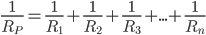9. What is Kirchoff's 2nd Law?
Kirchoff's 2nd Law states that the sum of the voltage drops around a circuit loop equals the sum of the voltage rises around the loop.
10. If 7A of current flow into a three way junction and 3A leave via one of the routes, how much current leaves via the other?
7A - 3A = 4A
11. What is the formula for capacitance?

C =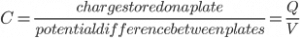12. What are the units of capacitance?
The units of capacitance are Farads.

13. What is the formula for the electric field between the plates of a capacitor?

E =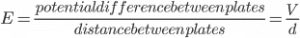14. (T/F) As plate area increases, capacitance increases proportionally.
True.
15. Does the introduction of a dielectric material between the plates of a capacitor increase or decrease the capacitance?
A dielectric material increases the capacitance.

The new capacitance (C') = CK, where K = dielectric constant.
16. What is the formula for calculating capacitance given capacitors in series?

Cs =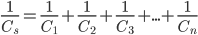17. What is the formula for calculating capacitance given capacitors in parallel?

Cp =
Cp = C1 + C2 + C3+ ... + Cn
18. For AC current, what is the formula for calculating Irms?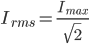19. For AC current, what is the formula for calculating Vrms?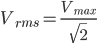Author: awc1990 ID: 212587 Card Set: Physics Flashcards - DC and AC Circuits Updated: 2013-04-10T05:43:09Z Folders: Description: Physics Flashcards - DC and AC Circuits Kaplan MCAT P42-P47 Show Answers: